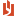## MS Mod 11 Single-Factor ANOVA Tukey’s procedure practice exam...

 Author Message NEASSupreme BeingGroup: Administrators Posts: 4.2K, Visits: 1.2K MS Module 11 Single-Factor ANOVA Tukey’s procedure practice exam questions(The attached PDF file has better formatting.)Five groups, each with 7 observations, have sample means of     1 = 3.2, 2 = 3.1, 3 = 3.5, 4 = 3.9, 5 = 3.7The total sum of squares (SST) is 20, and the treatment sum of squares (SSTr) is 11.The groups have normal distributions with equal variances. We test the null hypothesis μ1 = μ2 = μ3 = μ4 = μ5 at a 5% confidence level. If we reject the null hypothesis, we test which groups means differ significantly.Question 11.1: Error sum of squaresWhat is the error sum of squares SSE?Answer 11.1: 20 – 11 = 9(error sum of squares = total sum of squares – treatment sums of squares)Question 11.2: Treatment mean squareWhat is the mean square for the groups (treatment mean square)?Answer 11.2: 11 / (5 – 1) = 2.75(treatment mean square = treatment sums of squares / (number of groups – 1) )Question 11.3: Mean squared errorWhat is the mean squared error MSE?Answer 11.3: 9 / (5 × 7 – 5) = 0.30(degrees of freedom for mean squared error = (number of groups – 1) × observations per group; mean squared error = error sum of squares / its degrees of freedom)Question 11.4: F valueWhat is the F value to test the null hypothesis?Answer 11.4: 2.75 / 0.30 = 9.167Question 11.5: Critical F valueWhat is the critical F value for α = 5%?Answer 11.5: 2.69(Table look-up: α = 5%; degrees of freedom = 4, 30)Question 11.6: Critical Q valueWhat is the critical Q value for α = 5%?Answer 11.6: 4.10(Table look-up: α = 5%; degrees of freedom = 5, 30)Question 11.7: W (the width of the difference) for Tukey’s honestly statistical difference?What is w (the width of the difference) for Tukey’s honestly statistical difference?Answer 11.7: 4.1 × (0.30 / 7)0.5 = 0.849(Tukey’s W = critical Q value × (mean squared error / observations per group)0.5 ) AttachmentsMS Module 11 Single-Factor ANOVA Tukey’s procedure practice exam questions.pdf (171 views, 34.00 KB)
##### Merge Selected
Merge into selected topic...

Merge into merge target...

Merge into a specific topic ID...